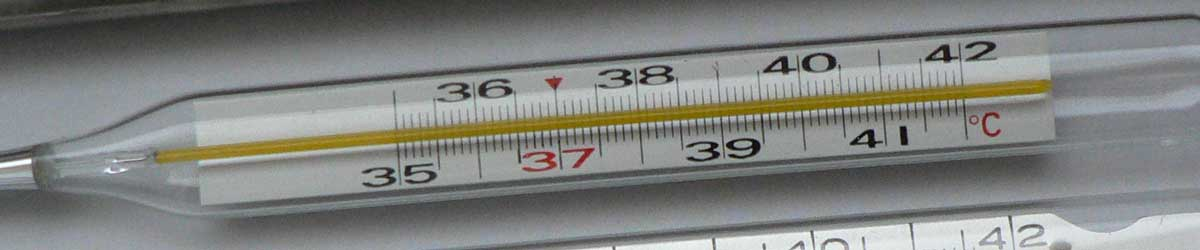# Newton to Kelvins Conversion

Enter the temperature in Newton below to get the value converted to kelvins.

Results in Kelvins:1 °N = 276.180303 K
Do you want to convert kelvins to Newton?

## How to Convert Newton to KelvinsConvert degrees Newton to kelvins with this simple formula:

kelvins = [°N] × 100 / 33 + 273.15

Insert the [°N] temperature measurement in the formula and then solve to find the result.

For example, let's convert 50 °N to kelvins:

50 °N = ( 50 × 100 / 33 + 273.15 ) = 424.665152 K

## Newton

The Newton scale is a temperature scale that sets a fixed zero point equal to the freezing point water. The scale uses both arithmetic progression and geometric progression. The scale defines 18 reference points and the corresponding "degrees of heat" and geometric progressions.

Newton can be abbreviated as N; for example, 1 degree Newton can be written as 1 °N.

## Kelvins

The Kelvin scale is an absolute temperature scale that uses absolute zero as the starting point. The kelvin is defined in terms of the Boltzmann constant, which is a measure of the energy of motion corresponding to one kelvin.

More specifically, one kelvin is equal to the change of thermodynamic temperature that results in a change of thermal energy kT by 1.380649 × 10−23 J.

0 kelvins are equal to −273.15 degrees Celsius, which is the point of absolute zero.

The kelvin is the SI base unit for temperature in the metric system. Kelvins can be abbreviated as K; for example, 1 kelvin can be written as 1 K.

The kelvin scale is different from other temperature scales in that it is not expressed in degrees, values are expressed in kelvins. When using the Kelvin scale in writing, the "k" is capitalized, however when using the kelvin as a unit the "k" should be lower-case, even though it is named for a person.

### Important Temperatures In Kelvins

TemperatureKelvins
Absolute Zero0 K
Freezing Point of Water273.15 K
Triple Point of Water273.16 K
Boiling Point of Water373.15 K

## Newton to Kelvin Conversion Table

Newton measurements converted to kelvins
Newton Kelvins
-90.14 °N 0 K
-50 °N 121.63 K
-40 °N 151.94 K
-30 °N 182.24 K
-20 °N 212.54 K
-10 °N 242.85 K
0 °N 273.15 K
10 °N 303.45 K
20 °N 333.76 K
30 °N 364.06 K
40 °N 394.36 K
50 °N 424.67 K
60 °N 454.97 K
70 °N 485.27 K
80 °N 515.57 K
90 °N 545.88 K
100 °N 576.18 K
110 °N 606.48 K
120 °N 636.79 K
130 °N 667.09 K
140 °N 697.39 K
150 °N 727.7 K
160 °N 758 K
170 °N 788.3 K
180 °N 818.6 K
190 °N 848.91 K
200 °N 879.21 K
300 °N 1,182 K
400 °N 1,485 K
500 °N 1,788 K
600 °N 2,091 K
700 °N 2,394 K
800 °N 2,697 K
900 °N 3,000 K
1,000 °N 3,303 K

## References

1. International Bureau of Weights and Measures, The International System of Units, 9th Edition, 2019, https://www.bipm.org/utils/common/pdf/si-brochure/SI-Brochure-9.pdf